# Ostrowski Type Inequalities for Higher-Order Derivatives

## Abstract

This paper has shown some new Ostrowski type inequalities involving higher-order derivatives. The results generalized the Ostrowski type inequalities. Applications of the inequalities are also given.

## 1. Main Result and Introduction

The following inequality is well known in literature as Ostrowski's integral inequality.

Letbe continuous onand differentiable onwhose derivativeis bounded on, that is,. Then(1.1)

Moreover the constant 1/4 is the best possible. Because Ostrowski's integral inequality is useful in some fields, many generalizations, extensions, and variants of this inequality have appeared in the literature; see  and the references given therein. The main aim of this paper is to establish some new Ostrowski type inequalities involving higher-order derivatives. The analysis used in the proof is elementary. The main result of this paper is the following inequality.

Theorem 1.1.

Suppose

(1)to be continuous on;

(2)to be nth order differentiable on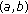whose nth order derivativeis bounded on, that is,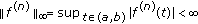;

(3)there exists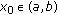such that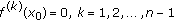.

Then for any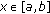, we have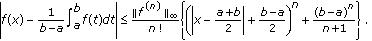(1.2)

As applications of the inequality (1.2), we give more Ostrowski type inequalities.

## 2. The Proof of Theorem 1.1

In this section, we use the Taylor expansion to prove Theorem 1.1. Before the proof, we need the following lemmas.

Lemma 2.1.

Suppose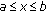and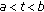, then we have(2.1)

Proof.

When, then(2.2)

When, then(2.3)

From (2.2) and (2.3), we know that (2.1) holds.

Lemma 2.2.

Suppose, then forwe have(2.4)

Proof.

It is obvious that (2.4) is true for. When, let(2.5)

then(2.6)

The only real root ofis. Notice(2.7)

Therefore we get the inequality (2.4).

Now, we give the proof of Theorem 1.1.

Proof.

Using the Taylor expansion ofatgives(2.8)

Taking the integral on both sides of (2.8) with respect to variableover, we have(2.9)

where the parameteris not a constant but depends on. From (2.8) and (2.9) one gets(2.10)

So we have(2.11)

Using Lemmas 2.1 and 2.2 gives (1.2). Thus, we complete the proof.

## 3. Some Applications

In this section, we show some applications of the inequality (1.2). In fact, we can use (1.2) to derive some new Ostrowski type inequalities.

Theorem 3.1.

Suppose

(1)to be continuous on;

(2)to be second order differentiable onwhose second derivativeis bounded on, that is,;

(3).

Then for any, we have(3.1)

Proof.

From Rolle's mean value theorem, we know that there existssuch that. Letin the inequality (1.2), then we have (3.1).

Corollary 3.2.

With the assumptions in Theorem 3.1, we have(3.2)

Proof.

For any, we have(3.3)

Consequently, (3.1) gives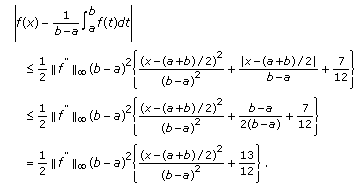(3.4)

Corollary 3.3.

With the assumptions in Theorem 3.1, we have(3.5)

Proof.

For any, we have(3.6)

Substituting (3.6) into (3.1) gives (3.5).

Theorem 3.4.

Suppose

(1)to be continuous on;

(2)to be nth order differentiable onwhose nth order derivativeis bounded on, that is,.

Then for anyand, we have(3.7)

Proof.

Let(3.8)

Then we have(3.9)

Using inequality (1.2) togives(3.10)

Since(3.11)

we have(3.12)

Using Ostrowski's integral inequality (1.1) one gets(3.13)

Notice(3.14)

Substituting (3.13) and (3.14) into (3.12) gives (3.7).

It is easy to see that (3.7) is the generalization of (1.2). If we letin (3.7) and use (3.6), we get the following inequality.

Corollary 3.5.

With the assumptions in Theorem 3.4, we have(3.15)

## References

1. Ostrowski A: Über die absolutabweichung einer differentienbaren Funktionen von ihren integralmittelwert. Commentarii Mathematics Helvetici 1938, 10: 226–227.

2. Anastassiou GA: Multivariate Ostrowski type inequalities. Acta Mathematica Hungarica 1997,76(4):267–278. 10.1023/A:1006529405430

3. Barnett NS, Dragomir SS: An Ostrowski type inequality for double integrals and applications for cubature formulae. RGMIA Research Report Collection 1998,1(1):13–22.

4. Dragomir SS, Barnett NS, Cerone P: An n-dimensional version of Ostrowski's inequality for mappings of the Hölder type. RGMIA Research Report Collection 1999,2(2):169–180.

5. Dragomir SS, Agarwal RP, Cerone P: On Simpson's inequality and applications. Journal of Inequalities and Applications 2000,5(6):533–579. 10.1155/S102558340000031X

6. Dragomir SS: Ostrowski type inequalities for isotonic linear functionals. Journal of Inequalities in Pure and Applied Mathematics 2002,3(5, article 68):1–13.

7. Florea A, Niculescu CP: A note on Ostrowski's inequality. Journal of Inequalities and Applications 2005, (5):459–468.

8. Pachpatte BG: On an inequality of Ostrowski type in three independent variables. Journal of Mathematical Analysis and Applications 2000,249(2):583–591. 10.1006/jmaa.2000.6913

9. Pachpatte BG: On a new Ostrowski type inequality in two independent variables. Tamkang Journal of Mathematics 2001,32(1):45–49.

## Acknowledgments

The author would like to express deep appreciation to the referees for the helpful suggestions. Mingjin Wang was supported by STF of Jiangsu Polytechnic University.

## Author information

Authors

### Corresponding author

Correspondence to Mingjin Wang.

## Rights and permissions

Open Access This article is distributed under the terms of the Creative Commons Attribution 2.0 International License (https://creativecommons.org/licenses/by/2.0), which permits unrestricted use, distribution, and reproduction in any medium, provided the original work is properly cited.

Reprints and Permissions

Wang, M., Zhao, X. Ostrowski Type Inequalities for Higher-Order Derivatives. J Inequal Appl 2009, 162689 (2009). https://doi.org/10.1155/2009/162689

• Revised:

• Accepted:

• Published:

• DOI: https://doi.org/10.1155/2009/162689

### Keywords

• Taylor Expansion
• Real Root
• Type Inequality
• Integral Inequality
• Ostrowski Type Inequality